Function Repository Resource:

# GeoSpatialDistance

Find the spatial distance between two points on Earth

Contributed by: Peter Cullen Burbery
 ResourceFunction["GeoSpatialDistance"][loc] finds the spatial distance between Here and loc. ResourceFunction["GeoSpatialDistance"][loc1,loc2] finds the spatial distance between locations loc1 and loc2 on Earth.

## Details

The spatial distance is the straight-line distance between two points on Earth, or the distance travelled if one could tunnel through the Earth from one point to the other.
The distance is returned in the unit system specified by \$UnitSystem.
To find the position of a location like a city or building with a string, use the resource function WikidataGeoPosition.

## Examples

### Basic Examples (3)

Find the spatial distance between your location and the North and South Poles:

 In:=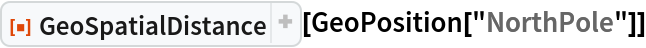Out=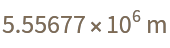In:=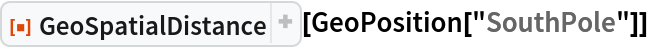Out=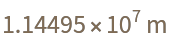Find the spatial distance between your location and its antipode:

 In:=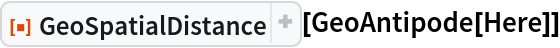Out=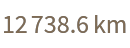Find the distance between New York City and Paris, France:

 In:=Out=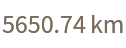### Applications (2)

The distance from Beijing to its antipode city:

 In:=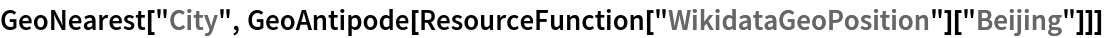Out=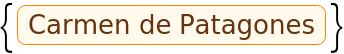In:=Out=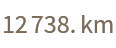Compare the direct distance to the geodesic distance:

 In:=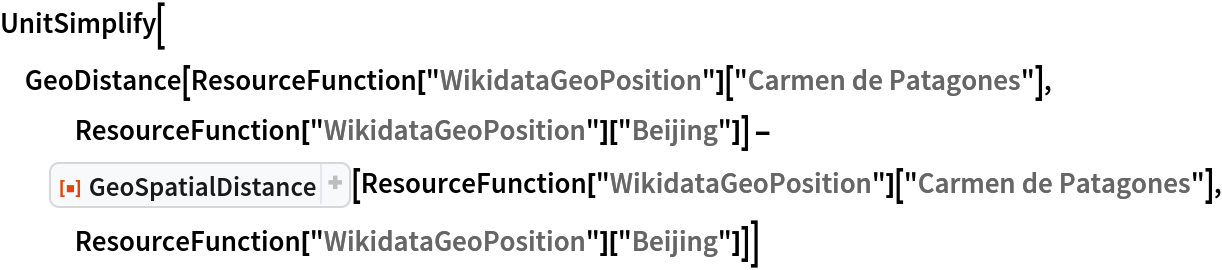Out=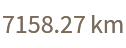### Properties and Relations (2)

Compare and contrast with GeoDistance:

 In:=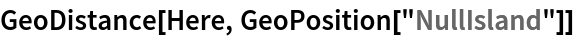Out=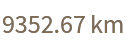In:=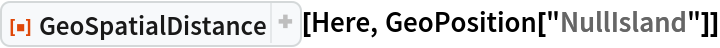Out=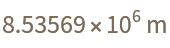In:=Out=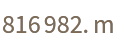Find the time it would take to travel at the speed of light to Null Island:

 In:=Out=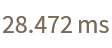Peter Burbery

## Version History

• 1.1.0 – 22 August 2022
• 1.0.0 – 16 August 2022

## Author Notes

I wanted to add an example with WikidataGeoPosition but its not functioning correctly right now. I was able to add an example with WikidataGeoPosition